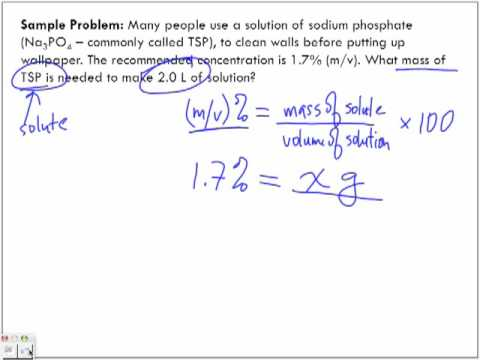What are some examples of percent concentration?

Aug 24, 2014

Here are three examples of percent concentration.

PERCENT BY MASS (m/m)

Percent by mass (m/m) is the mass of solute divided by the total mass of the solution, multiplied by 100 %.

Percent by mass = $\text{mass of solute"/"mass of solution}$ × 100 %

EXAMPLE

What is the percent by mass of rubbing alcohol in a solution that contains 275 g of rubbing alcohol in 500 g of solution?

Solution

Percent by mass = "mass of rubbing alcohol"/"mass of solution" × 100 % = (275"g")/(500"g") × 100 % =
55.0 % (m/m)

PERCENT BY MASS OVER VOLUME (m/v)

Percent (m/v) is the mass of solute divided by the volume of the solution, multiplied by 100 %.

Percent (m/v) = $\text{mass of solute"/"volume of solution}$ × 100 %

EXAMPLE

If the density of the above solution is 0.857 g/mL, what is the percent (m/v) of rubbing alcohol?

Solution

Volume of solution = 500 g solution × $\left(1 \text{mL solution")/ (0.857"g solution}\right)$ = 583.4 mL solution
(3 significant figures + 1 guard digit)

Percent (m/v) = "mass of rubbing alcohol"/"volume of solution" × 100 % = (275"g")/(583.4"mL") × 100 % = 47.1 %

PERCENT BY VOLUME (v/v)

Percent by volume (v/v) is the volume of solute divided by the total volume of the solution, multiplied by 100 %.

Percent by volume = $\text{volume of solute"/"total volume of solution}$ × 100 %

EXAMPLE

What is the percent by volume in a solution that contains 350 mL of rubbing alcohol 500 mL of solution?

Solution

Percent by volume = $\text{volume of rubbing alcohol"/"volume of solution}$ × 100 % = $\left(350 \text{mL")/(500"mL}\right)$ × 100 % = 70.0 % (v/v)